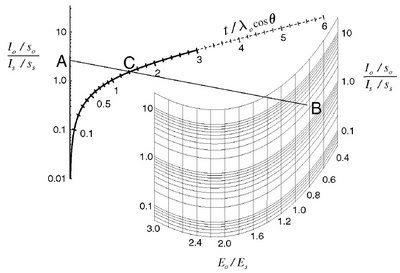### The Thickogram

Peter Cumpson at NPL in the United Kingdom has developed a useful graphical method for measuring overlayer thickness in samples where the overlayer has a different elemental chemistry than the substrate (for example: a niobium film over a silicon substrate). The method allows for the following:

1) Uniform surface contamination (e.g. adventitious carbon layer) is not important.
2) Unknown instrumental factors common to substrate and oxide cancel out.
3) Uses a simple equation.
4) Works well for large and small film thickness.

Some rules:
1) Emission angle must be between 0 and 60 degrees (ie. Take-off angle of 90 to 30 degrees). Emission angles around 45 degrees are the most accurate.
2) Applicable to a wide range of Kinetic Energies above ~500 eV.
3) Error in result is +/-10% based on accuracy of attenuation lengths obtained by calculations.

Values Needed: o = Overlayer, s= Substrate
Io = Intensity of Overlayer Peak (or Peak Area)
Is = Intensity of Substrate Peak (or Peak Area)
So = R.S.F. of Overlayer Peak
Ss = R.S.F. of Substrate
Eo = K.E. of Overlayer Peak
Es = K.E. of Substrate Peak
Theta = emission angle, (0 for 90 degree take-off)
Cos(Theta) (=1 for 90 degree take-off)
Lambda o = attenuation length of photoelectrons (from the overlayer) in the overlayer.

How to Use It: A printable version of the Thickogram (Figure 1) can be downloaded here. An Excel spreadsheet that is useful for multiple calculations is presented here. (Note: you must download the file to Excel to use it - it is locked in Google Docs).
1) Calculate A = Io/So / Is/So, add point on Thickogram
2) Calculate B = Eo/Es, add point on Thickogram
3) Draw a line from A to B. Point C is found on the curve.
4) Thickness (t) is calculated as t = C(Lambda o)cos(Theta)Figure 1. The Thickogram showing points A, B and C.
If you wish to use this method I would highly recommend reading through the original paper first .

Reference:
 Peter J. Cumpson, Surf. Interface Anal. 29, 403-406 (2000).